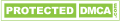# Basic Knowledge of Thermocouple

Thermocouple is a temperature sensing element, a kind of instrument which can measure temperature directly and turn temperature signal to emf signal, and transformed into the temperature of the measured medium through electrical instrument (secondary instrument).

The basic principle of thermocouple temperature measurement is that two kinds of conductors made of different materials constitute a closed loop circuit, when there is a temperature gradient between both ends, there will be a current passing through, and then, there will be electromotive force-thermoelectric emf existed on both ends, this is the so-called Seebeck Effect.

Two different components of homogeneous conductor electrode are thermodes, the end at which temperature is higher is the work end, and the other end at which temperature is lower is the free end which is usually under a constant temperature.

According to the function relationship between thermoelectric emf and temperature, we made the thermocouple indexing table when the temperature of free end is at 0 ℃, and different thermocouples has different indexing table.

When switching the third metal material in thermocouple loop, once the two contacts at the same temperature, the thermoelectric force produced by the thermocouple will remain the same, which means it wouldn’t be affected by the third metal switching in the loop. Therefore, you can connect the measuring instrument when using the thermocouple measure temperature, after you measured the thermoelectric emfs, then you can know the temperature of the medium measured.

Related Articles